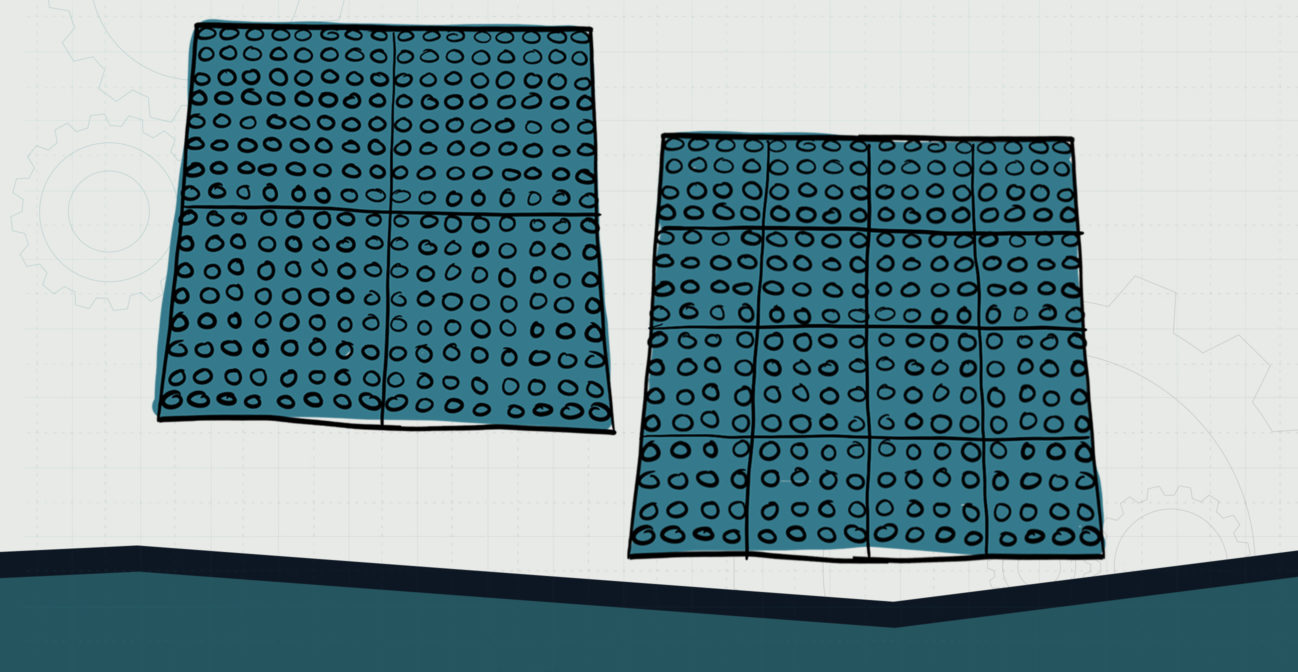# Divide a Build

## Explore division by breaking brick plates into smaller bricks.Objective:
Child will be able to understand the basics of division.

Essential Question(s):
How can we better understand division of large groups of numbers?

Special Materials:
None

Bricks Required:
16×16 plates, plates of other sizes (not 1×1 or 1×2)

Project Structure

Engage/Explore

1. Ask child to use smaller plates to divide up a 16×16 plate by laying them next to each other and counting how many it takes to cover the whole plate.
2. Ask: “How many small plates did it take to divide the larger plate?” “Do you have any remainder or studs left over?”
3. Have child write out a division problem to reflect the model in front of them.

Explain

1. Ask child to repeat the process above using a different-sized brick to cover the plates.
2. Have child model this division problem as well, and ask them how it differs from the previous one.
3. Child then calculates how many studs are on each plate, and writes a division sentence to show their math.
4. Have child compare their model to the written math problems to check their work.